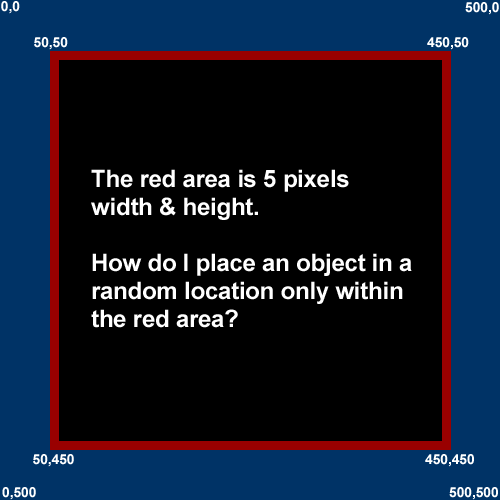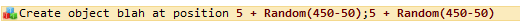# Placing an object within a specific area (math problem)

My question is in the box graphic below, and my code is below that.

Thank you for your help!My wrong code.It’s “50 +” and “50” instead of “5 +” and “5” (why have you put 5 ?)

Surely Victor, but more precisely, the init area in both axis is: 50+5 = 55, and the width/height is: 450-50-5-5 = 390 (if the red edges aren’t included)

``55 + Random(390)``

If you want the entire object get inside the area:

``X = 55 + Random(390 - Object.Width())``
``Y = 55 + Random(390 - Object.Height())``

Thank you both for your help. I appreciate it!